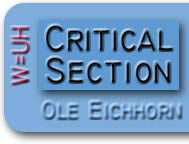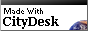Home

I hold the following to be the "equation of life":

W=UH

Where W=wrongness, U=ugliness, and H=hardness.  In English this equation means:

"if something is ugly or hard, it is wrong"

I first formulated this philosophy in regards to programming computers, where it is undoubtedly true, but gradually realized it had applicability to life in general.

You may have noticed the domain for this site is w-uh.com.  Well, I would have used w=uh.com, but equal signs aren't valid in a domain name.  Then I noticed that  if W=UH then W-UH=0, so w-uh is not only close, it is equivalent.  Kind of pretty, so it must be, er, right.  A recursive example of W=UH in action!